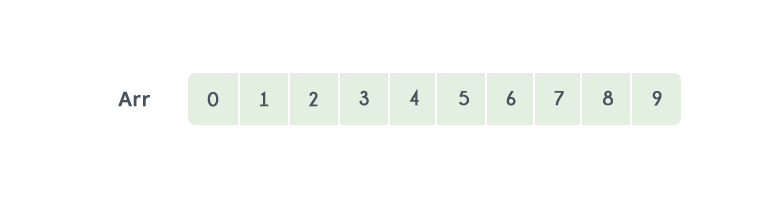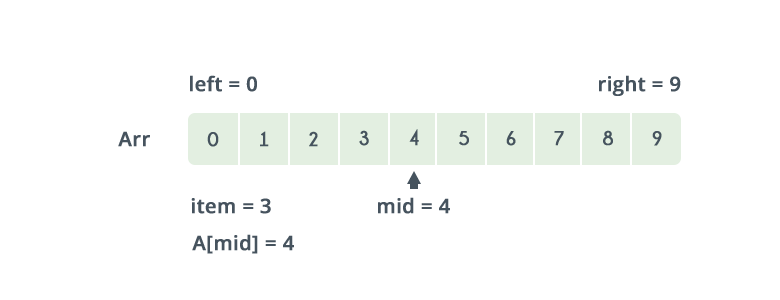Algorithms
Topics:
Binary Search

# Binary Search

Binary search is the most popular Search algorithm.It is efficient and also one of the most commonly used techniques that is used to solve problems.

If all the names in the world are written down together in order and you want to search for the position of a specific name, binary search will accomplish this in a maximum of $35$ iterations.

Binary search works only on a sorted set of elements. To use binary search on a collection, the collection must first be sorted.

When binary search is used to perform operations on a sorted set, the number of iterations can always be reduced on the basis of the value that is being searched.

Let us consider the following array:By using linear search, the position of element 8 will be determined in the $9^{th}$ iteration.

Let's see how the number of iterations can be reduced by using binary search. Before we start the search, we need to know the start and end of the range. Lets call them Low and High.

Low = 0
High = n-1


Now, compare the search value $K$ with the element located at the median of the lower and upper bounds. If the value $K$ is greater, increase the lower bound, else decrease the upper bound.Referring to the image above, the lower bound is $0$ and the upper bound is $9$. The median of the lower and upper bounds is (lower_bound + upper_bound) / 2 = 4. Here a = 4. The value 4>2, which is the value that you are searching for. Therefore, we do not need to conduct a search on any element beyond 4 as the elements beyond it will obviously be greater than 2.

Therefore, we can always drop the upper bound of the array to the position of element 4. Now, we follow the same procedure on the same array with the following values:

Low:   0
High:  3


Repeat this procedure recursively until Low > High. If at any iteration, we get $a[mid]= key$, we return value of $mid$. This is the position of $key$ in the array. If $key$ is not present in the array, we return $-1$.

Implementation:

int binarySearch(int low,int high,int key)
{
while(low<=high)
{
int mid=(low+high)/2;
if(a[mid]<key)
{
low=mid+1;
}
else if(a[mid]>key)
{
high=mid-1;
}
else
{
return mid;
}
}

As we dispose off one part of the search case during every step of binary search, and perform the search operation on the other half, this results in a worst case time complexity of $O(log _{2} N)$.